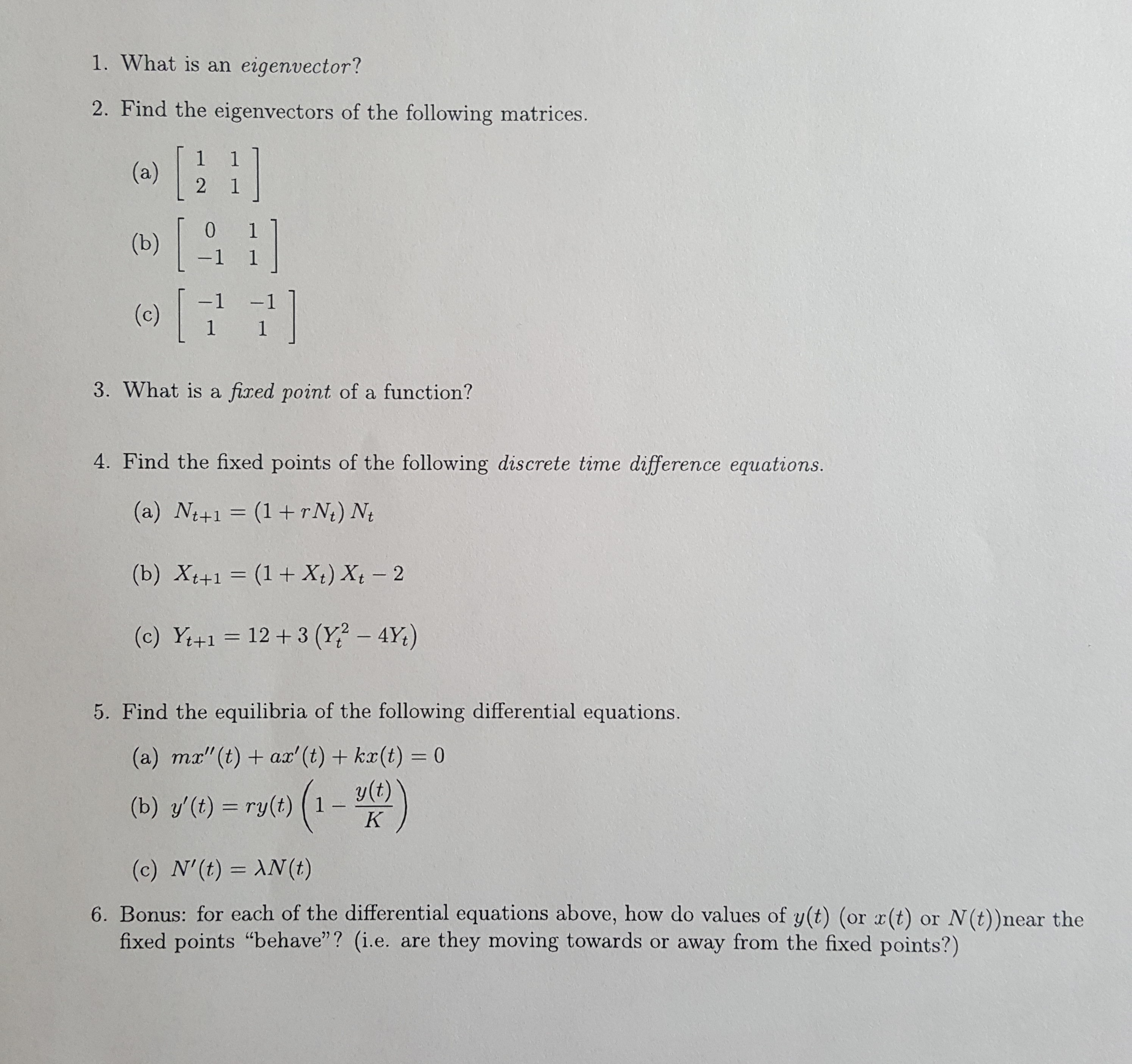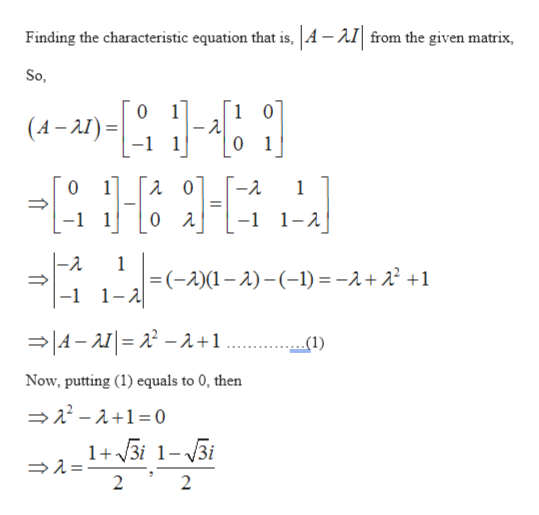1. What is an eigenvector?2. Find the eigenvectors of the following matrices.(a)21[0(b)-1 1-1(c)1 13. What is a fixed point of a function?4. Find the fixed points of the following discrete time difference equations.(a) Nt+1 (1+rN) Nt(b) Xt+1 (1 + X) Xt 212+3 (Y? - 4Y)(c) Y+15. Find the equilibria of the following differential equations.(a) mx"(t) ax' (t)+ kx(t) = 0y(t)1(b) y'(t) ry(t)K(c) N'(t) AN (t)6. Bonus: for each of the differential equations above, how do values of y(t) (or x(t)fixed points "behave"? (i.e. are they moving towards or away from the fixed points?)or N(t))near the

Question

I need help for problem 2b. Thankshelp_outlineImage Transcriptionclose1. What is an eigenvector? 2. Find the eigenvectors of the following matrices. (a) 2 1 [ 0 (b) -1 1 -1 (c) 1 1 3. What is a fixed point of a function? 4. Find the fixed points of the following discrete time difference equations. (a) Nt+1 (1+rN) Nt (b) Xt+1 (1 + X) Xt 2 12+3 (Y? - 4Y) (c) Y+1 5. Find the equilibria of the following differential equations. (a) mx"(t) ax' (t)+ kx(t) = 0 y(t) 1 (b) y'(t) ry(t) K (c) N'(t) AN (t) 6. Bonus: for each of the differential equations above, how do values of y(t) (or x(t) fixed points "behave"? (i.e. are they moving towards or away from the fixed points?) or N(t))near the fullscreen
Step 1

Given: -

Step 2

To calculate: -

Eigen vectors of the given matrix.

Step 3

Calculation...help_outlineImage TranscriptioncloseFinding the characteristic equation that is, |A -AI from the given matrix So, 1 0 1 0 (A-I) 1 0 1 0 -2 1 -1 1- 0 A -2. 1 =(-2)(1-)-(-1) = -2+22 +1 -1 1-2 A-A=22-2+1 (1) Now, putting (1) equals to 0, then 2-2+1 0 1+3i 1-3i 2 2 fullscreen

Want to see the full answer?

See Solution

Want to see this answer and more?

Our solutions are written by experts, many with advanced degrees, and available 24/7

See Solution
Tagged in

Matrices